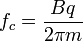Home » Uncategorized » Why a cyclotron cannot be used to accelerate an electron?

Why a cyclotron cannot be used to accelerate an electron?

why a cyclotron cannot be used to accelerate an electron?

The mass of electron is very less. So, when it is accelerated in a cyclotron, its speed increases rapidly and reaches relativistic values. (i.e. speeds comparable to the speed of light) Then the mass of the electron increases according to the equation,

This change in mass changes the frequency of revolution of electron. SinceTherefore the electron goes out of phase with the cyclotron frequency and acceleration stops.

This happens  because as the relativistic mass increases due to increase in speed, the frequency decreases which means that the time period of revolution increases. Therefore the polarity of the Dees reverses before the electron completes half revolution and the accelerator will not function properly.

Visitors So Far @ AskPhysics

• 2,156,001 hits

Subscribe to Blog via EmailAsk Physics